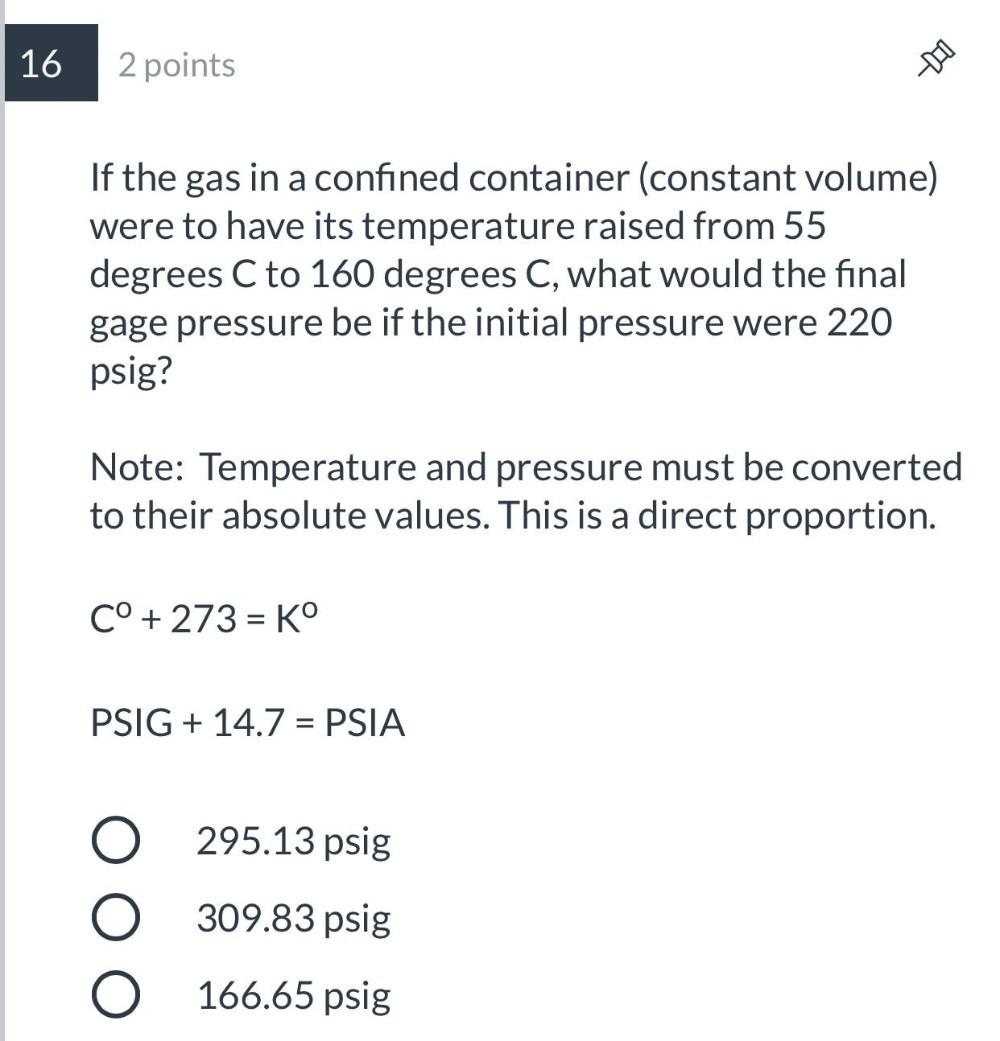Question:

# If the gas in a confined container (constant volume) were to have its temperature raised from 55 degrees C to 160 degrees C, what would the final gage pressure be if the initial pressure were 220 psigIf the gas in a confined container (constant volume) were to have its temperature raised from 55 degrees C to 160 degrees C, what would the final gage pressure be if the initial pressure were 220 psig? Note: Temperature and pressure must be converted to their absolute values. This is a direct proportion. C° +273 = Kº PSIG + 14.7 = PSIA (A)295.13 psig (B)309.83 psig (C)166.65 psig